# Slope form

Find the equation of a line given the point X(8, 1) and slope -2.8. Arrange your answer in the form y = ax + b, where a, b are the constants.

a =  -2.8
b =  23.4

### Step-by-step explanation: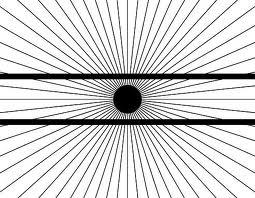Did you find an error or inaccuracy? Feel free to write us. Thank you!Tips to related online calculators
Line slope calculator is helpful for basic calculations in analytic geometry. The coordinates of two points in the plane calculate slope, normal and parametric line equation(s), slope, directional angle, direction vector, the length of the segment, intersections of the coordinate axes, etc.

## Related math problems and questions:

• V - slopeThe slope of the line whose equation is -3x -9 = 0 is
• General line equationsIn all examples, write the GENERAL EQUATION OF a line that is given in some way. A) the line is given parametrically: x = - 4 + 2p, y = 2 - 3p B) the line is given by the slope form: y = 3x - 1 C) the line is given by two points: A [3; -3], B [-5; 2] D) t
• Line segmentFor the line segment whose endpoints are L[-1, 13] and M[18, 2], find the x and y value for the point located 4 over 7 the distance from L to M.
• LineWrite an equation of a line parallel to To 9x + 3y = 8 That Passes Through The Point (-1, -4). Write in form ax+by=c.
• Slope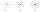Calculate the slope of a line that intersects points (-84,41) and (-76,-32).
• Find the 3Find the distance and midpoint between A(1,2) and B(5,5).
• SlopeWhat is the slope of the line defined by the equation -2x +3y = -1 ?
• Using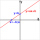Using the point-slope equation, find the equation containing (-7, 3) and slope m = -4
• Points on line segmentPoints P & Q belong to segment AB. If AB=a, AP = 2PQ = 2QB, find the distance: between point A and the midpoint of the segment QB.
• Perpendicular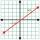What is the slope of the perpendicular bisector of line segment AB if A[9,9] and B[9,-2]?
• The midpointThe midpoint of (2, 5) and (8, y) is (5, -1). Find the line equation in slope-intercept form.
• The coordinates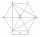The coordinates (5, 2) and (-6, 2) are vertices of a hexagon. Explain how to find the length of the segment formed by these endpoints. How long is the segment?
• Lie/do not lie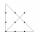The function is given by the rule f(x) = 8x+16. Determine whether point D[-1; 8] lies on this function. Solve graphically or numerically and give reasons for the your answer.
• SlopeFind the slope of the line: x=t and y=1+t.
• Perpendicular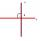Determine the slope of the line perpendicular to the line p: y = -x +4.
• Midpoint of segmentPoint A has coordinates [4; -11] and the midpoint of the segment AB is the point [17; -7]. What are the coordinates of point B?
• Points OPQ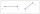Point P is on line segment OQ. Given OP = 6, OQ = 4x - 3, and PQ = 3x, find the numerical length of OQ.